Function Repository Resource:

# NewtonCotesError

Find the error in the Newton–Cotes quadrature formula

Contributed by: Jan Mangaldan
 ResourceFunction["NewtonCotesError"][n,f,a,b] gives the error in the elementary n-point Newton–Cotes quadrature formula for the function f on an interval from a to b.

## Details and Options

The function f may be a pure function or a named function defined using Set or SetDelayed.
The error is given as a function of n, a and b multiplied by an mth derivative of the function f. The size of the error is bounded by the maximum of this expression over the interval from a to b.
The abscissas may or may not include the endpoints a and b of the interval. The option "QuadratureType" is used to control whether endpoints are included as abscissas.
With the default setting "QuadratureType"Closed, the endpoints are included as abscissas.
With the setting "QuadratureType"Open, the endpoints are not included as abscissas. In this case, the initial abscissa is chosen to be a half step above the lower endpoint.

## Examples

### Basic Examples (1)

Find the error in the five-point Newton–Cotes quadrature of a function:

 In:=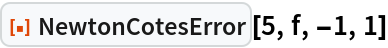Out=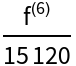### Scope (2)

Determine the error in the Newton–Cotes approximation with four steps between 2 and 7 for a pure function:

 In:=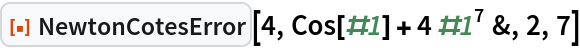Out=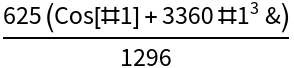The error for a function defined using SetDelayed: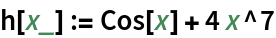In:=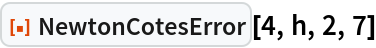Out=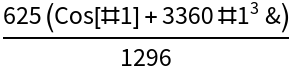### Properties and Relations (2)

The Newton–Cotes error involves a derivative of f at an unknown point, so you do not really know what the error itself is:

 In:=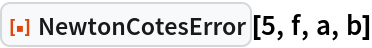Out=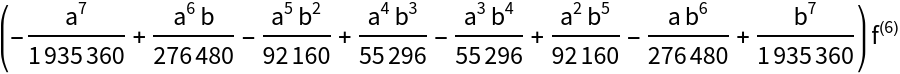However, the error decreases rapidly with the length of the interval:

 In:=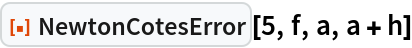Out=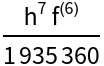For a polynomial of order n, the n-point Newton–Cotes formula gives zero error, expressed through a zero-valued function 0&: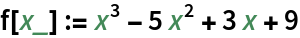In:=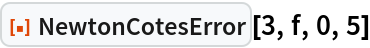Out=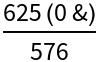The Newton–Cotes result is the same as the one returned by Integrate:

 In:=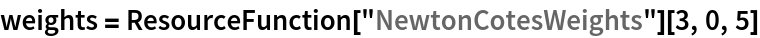Out=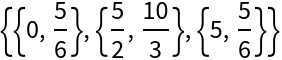In:=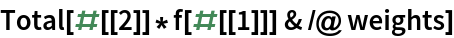Out=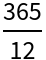In:=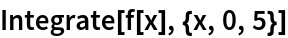Out=## Version History

• 1.1.0 – 04 January 2021
• 1.0.0 – 15 November 2019